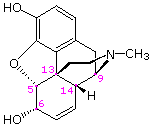# How many chiral centers are in morphine?

Jun 5, 2015

There are five chiral centres in morphine.

#### Explanation:

The systematic name of morphine is ($1 S , 5 R , 13 R , 14 S , 17 R$)-4-methyl-12-oxa-4-azapentacyclo[$9.6 .1 {.0}^{1 , 13} {.0}^{5 , 17} {.0}^{7 , 18}$]octadeca-7(18),8,10,15-tetraene-10,14-diol.

Now you know why we just call it morphine!

Its structure isThe five atoms with the wedges and dashes are the chiral centres:

• $\text{C-5}$, the carbon joined to the epoxide
• $\text{C-6}$, the carbon attached to the $\text{OH}$ group
• $\text{C-9}$, the carbon attached to $\text{N}$
• $\text{C-13}$, the carbon at the bridgehead of four different rings
• $\text{C-14}$, the carbon at the bridgehead of two rings